# Active Learning on MNIST – saving on labeling

Active Learning is a semi-supervised technique that allows to label less data by selecting most important samples from the learning process (loss) standpoint. This can have a huge impact on the cost of the projects in the case when the amount of data is large and labeling rate is high. For example for object detection and NLP-NER problems.

The article is based on the code: Active Learning on MNIST

# Data for the experiment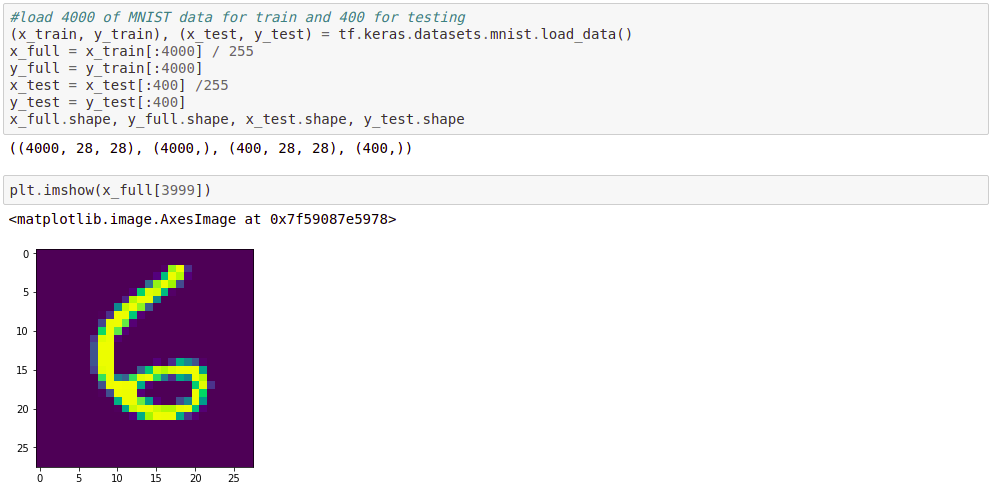I will use a subset of MNIST dataset that is 60K pictures of digits with labels and 10K test samples. For the purposes of quicker training I will use 4000 samples (pictures) for training and 400 for test (neural network will never see it during the training). For normalization I divide the grayscale image points by 255.

# Model, training and labeling processes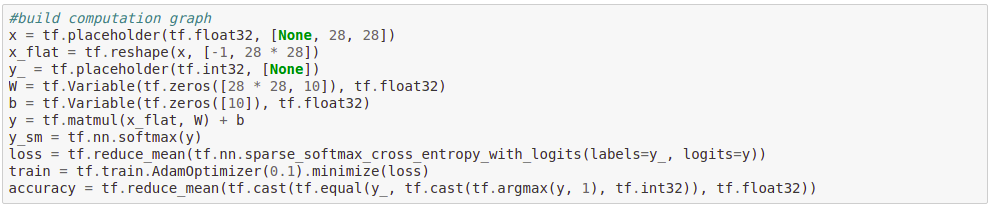As a framework i will use TensorFlow computation graph that will build ten neurons (for every digit). W and b are weights for the neurons. I will need a softmax output y_sm for probabilities (confidence) of digits. The loss will be a typical “softmaxed” cross entropy between predicted and labeled data. Choice for the optimizer is a popular Adam, learning rate is almost default – 0.1. As a main metric I will use accuracy over test dataset.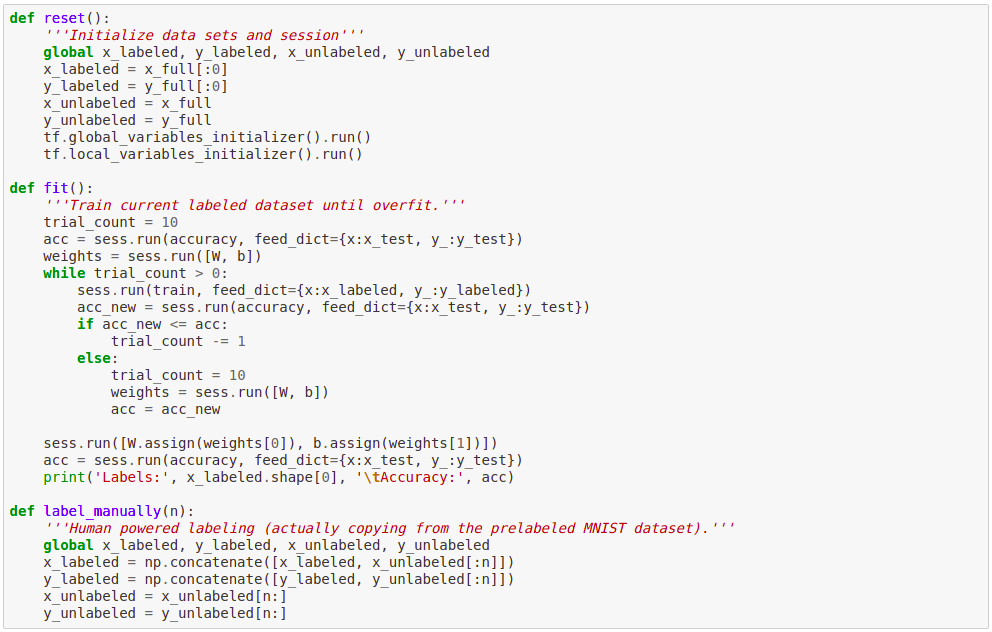I am defining three procedures for more convenient coding.
reset() – empties the labeled dataset, puts all data in unlabeled dataset and resets the session variables
fit() – run a training attempting to reach the best accuracy. If for ten attempts it cannot improve – the training stops on the previous best result. We cannot use just some big number of trainings epochs as the model tends quickly to overfit or needs an intensive L2 regularization.
label_manually() – this is an emulation of human data labeling. Actually we take the labels from the MNIST dataset that is labeled already.

# Ground Truth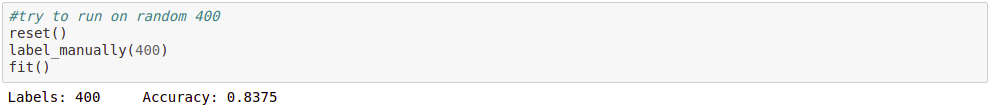If we would be so lucky to have enough resources to label whole dataset, we will receive the 92.25% of accuracy

# ClusteringHere I try to use k-means clustering to find group of digits and that use this information for automatic labeling. I run tensorflow clustering estimator and then visualize the resulting ten centroids. As you can see the result if far from perfect – digit “9” is represented three times, sometimes mixed with “8” and “3”.

# Active Learning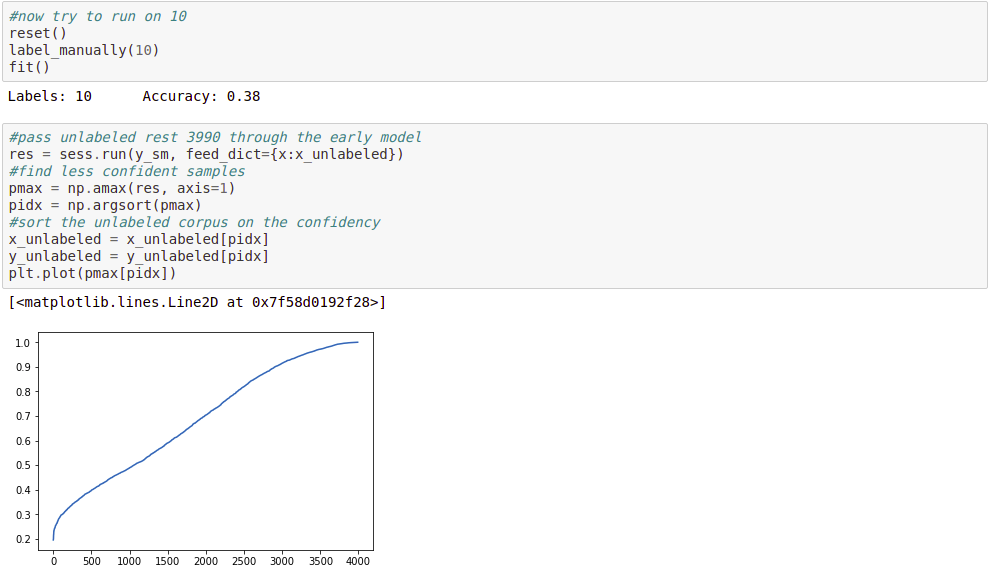Now we will label the same 10% of data (400 samples) using active learning. We will take one batch of 10 samples and train a very primitive model. Then will pass rest of data (3990 samples) through this model and evaluate the maximum softmax output, which is the probability of the selected class to be the correct answer (confidence of the neural network). After sorting we can see on the plot that the distribution of confidence vary from 20% to 100%. The idea is to select next batch for labeling exactly from the LESS CONFIDENT samples.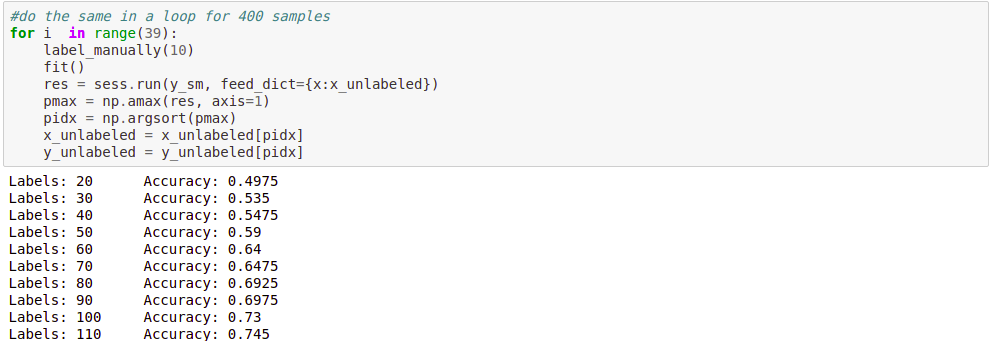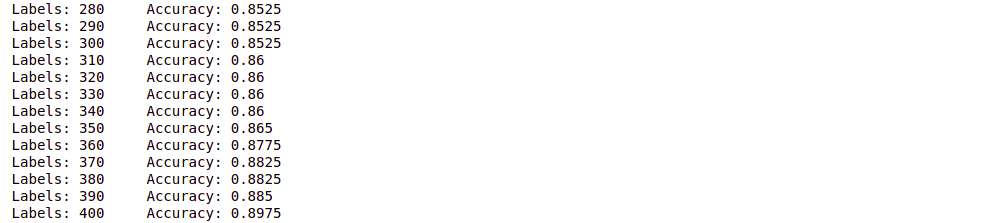After running such procedure for 40 batches of 10 samples we can see that the resulting accuracy is almost 90%, what is far more than 83.75% in the random labeled data case.

# What to do with the rest of unlabeled data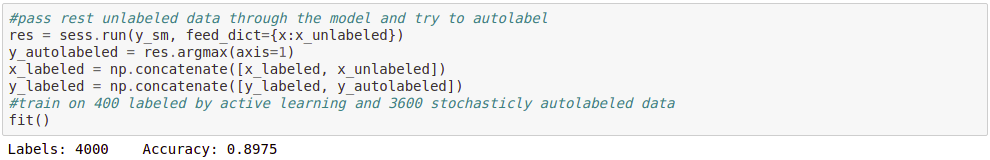Classical way is to run the rest of the dataset through the existing model and automatically label the data. Then push it in the training process and maybe it will help to better tune the model. In out case this did not give us any better result.
My approach is to do the same but, as in the active learning, taking in consideration the confidence:Here we run the rest of unlabeled data through the model evaluation and we still can see that the confidence differs for the rest of samples. Thus the idea is to take a batch of ten MOST CONFIDENT samples and train the model.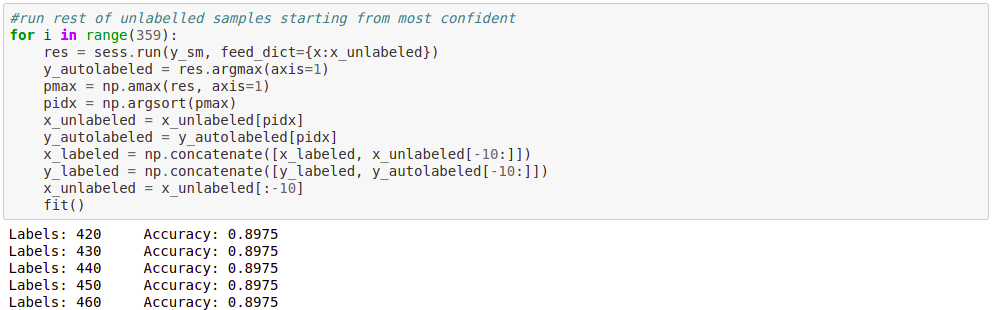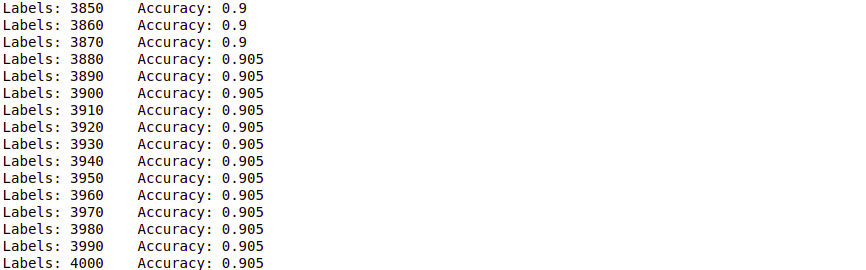This process takes some time and gives us extra 0.8% of accuracy.

# Results

Experiment Accuracy
4000 samples 92.25%
400 random samples 83.75%
400 active learned samples 89.75%
+ auto-labeling 90.50%

# Conclusion

Of course this approach has drawbacks in form of intensive usage of computation resources and requires special procedure for data labeling mixed with early model evaluation. Also for the testing purposes data needs to be labeled as well. However if the cost for a label is high (especially for NLP, CV projects), this method can save significant amount of resources and make the project results better.

Posted on Categories Craft